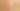RightToLeftChaining

Misconception:

When chaining methods or fields, like with a().b(), a.b(), a().b or a.b, members are accessed from right to left (first the right most member). Also when an object instantiation is involved , e.g., new C().a(), the right member of a chaining operation does have precedence over the object instantiation (new C()). Therefore statements such as new C().a() are invalid: valid code is instead (new C()).a().Incorrect

Chained accesses are invoked from right to left

Correct

Chained accesses are invoked from left to right

CorrectionHere is what's right.

The dot operator is left-associative: a.b.c is equivalent to (a.b).c. This implies that chained operations are evaluated from left to right.

The following two code snippets both invoke method a() and then invoke method b() on the object returned by method a():

T o = a();
o.b();
a().b()

Here we see a correct expression tree for the above expression:

The correct way to execute chained method calls, is from left to right (from the bottom to the top in the expression tree). For the example expression, first a() is called, then b() is called on the return value of a(). The fact that b() is called on the return value of a() implies that a() has to be called before b(), and thus that these calls evaluate from left to right.

This misconception applies to method calls (like o.a().b()), field accesses (like o.f.g), and any mix thereof (e.g., o.a().f.b().h).

SymptomsHow do you know your students might have this misconception?

This misconception can manifest itself when drawing expression trees, e.g., the tree for a().b(), where students mistakenly construct the tree top-down (here, an a() node above a b() node).

Java